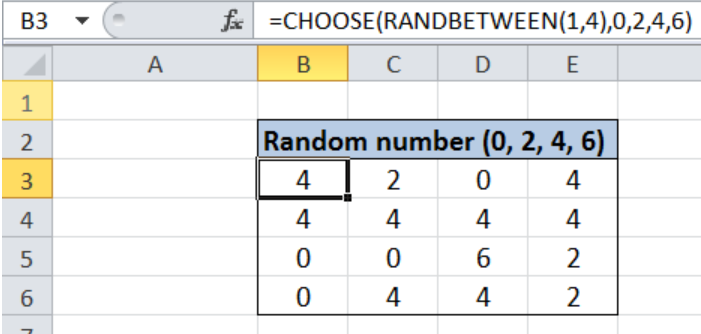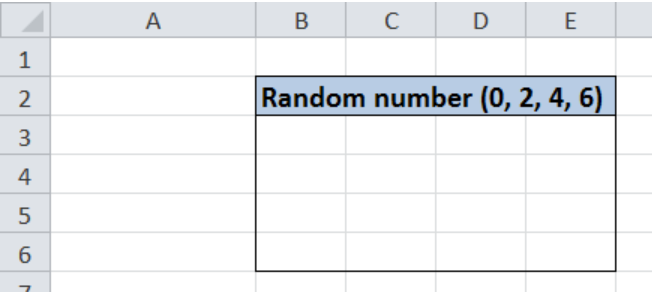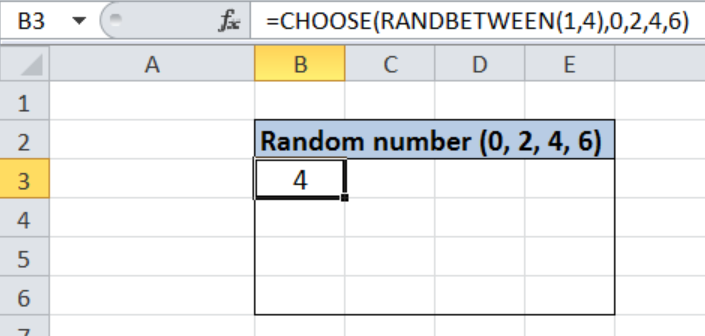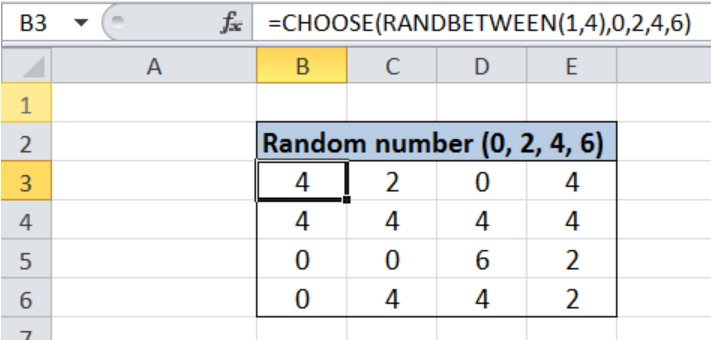Get instant live expert help with Excel or Google Sheets“My Excelchat expert helped me in less than 20 minutes, saving me what would have been 5 hours of work!”

#### Post your problem and you’ll get Expert help in seconds.

Your message must be at least 40 characters
Our professional Expert are available now. Your privacy is guaranteed.

# Random number from fixed set of options

While working with Excel, we are able to generate random numbers by using the RANDBETWEEN function.  However, if we have a given set of values to choose from, we can combine the CHOOSE and RANDBETWEEN functions.  This step by step tutorial will assist all levels of Excel users in generating random numbers from a fixed set of options.Figure 1. Final result: Random number from fixed set of options

Final formula: `=CHOOSE(RANDBETWEEN(1,4),0,2,4,6)`

## Syntax of CHOOSE Function

CHOOSE function returns a value from a list of values based on the index_num provided

`=CHOOSE(index_num, value1, [value2], ...)`

• index_num  – determines which value in the list of values is returned by the CHOOSE function
• Index_num must be a number between 1 and 254, or a cell reference with values between 1 and 254
• Value1 is returned if index_num is 1, values2 if index_num is 2; and so on
• CHOOSE returns the error #VALUE! if index_num is less than 1 or greater than the number of the last value in the list
• value1, value2, …   Only value 1 is required, succeeding values are optional;  The arguments can be numbers, cell references, defined names, formulas, functions, or text.

## Syntax of RANDBETWEEN Function

RANDBETWEEN randomly returns an integer between the numbers we specify

`=RANDBETWEEN(bottom, top)`

• bottom  – the smallest integer that RANDBETWEEN will randomly return
• top  – the largest integer that RANDBETWEEN will randomly return

## Setting up Our Data

Here we have a 4×4 table which we will randomly populate with the numbers 0, 2, 4 and 6.Figure 2. Sample data for random number from fixed set of options

## Generate random number

We want to fill cells B3:E6 with the numbers 0, 2, 4 and 6.  We can do this by using the CHOOSE and RANDBETWEEN functions.  Here are the steps:

Step 1.  Select cell B3

Step 2.  Enter the formula: `=CHOOSE(RANDBETWEEN(1,4),0,2,4,6)`

Step 3:  Press ENTER

Step 4:  Copy the formula in cell B3 to cells B3:E6 by clicking the “+” icon at the bottom-right corner of cell B3 and dragging it down to B6, then right towards E6.Figure 3. Entering the formula using CHOOSE and RANDBETWEEN

Our formula chooses a number from a fixed set of options: 0, 2, 4 and 6.  There are four options, so the index_num for our CHOOSE function should be an integer between 1 and 4.  The index_num is determined by the function: RANDBETWEEN(1,4). The RANDBETWEEN generates random integers from 1 to 4, which in turn determines which number CHOOSE returns.

For index_num 1, CHOOSE will return the value “0”; for index_num 2, it will return “2”; for index_num 3, it will return “4”; and for index_num 4, it will return “6”.

Below table shows our 4×4 table already filled randomly with numbers 0, 2, 4 and 6.Figure 4. Output: Random number from fixed set of options

Note: The random integer number returned by RANDBETWEEN changes every time the worksheet is changed.  We can fix the values to prevent further changes by following these steps:

Step 1.  Select cells B3:E6

Step 2.  Press Ctrl + C to copy the values

Step 3:  Right click then choose “Values (V)” under the Paste Options

Step 4:  Press Enter

This will change the formulas into values and prevent any further random changes.

Most of the time, the problem you will need to solve will be more complex than a simple application of a formula or function. If you want to save hours of research and frustration, try our live Excelchat service! Our Excel Experts are available 24/7 to answer any Excel question you may have. We guarantee a connection within 30 seconds and a customized solution within 20 minutes.

### Did this post not answer your question? Get a solution from connecting with the expert.Another blog reader asked this question today on Excelchat:
Related blogs
Solution examplesI need a formula that has these 2 rules. If letter was rec'd between 1st and 15th = 1st of the month following letter rec'd date. If letter was rec'd between 16thst and end of the month= 1st of the 2nd month following letter rec'd date.
Solved by V. F. in 20 minsI need a column to total (Hours)(85) + (Travel)(.55) per line
Solved by F. Q. in 20 minsTrying to figure out what is wrong with my formula in Oct-ZD tab in column AY and BG.
Solved by O. Q. in 8 minsSo I have used vlookup to find the price of a product on a different sheet and then I have tried to times that by a quantity. e.g. =vlookup(d4,table35,2,false)*e4 This is the formula I typed in which worked for another list I did but for some reason it's not working now even though everything is practically the same, apart from the contents. Could you help?
Solved by B. U. in 60 minsI have a column containing date and time data together and i need to isolate the time data. When I tried to use text to column, it changed all the times to AM. There is an extremely large amount of data - over 550,000 rows so manually changing it won't work. The file is too large to attach.
Solved by S. E. in 40 mins## Subscribe to Excelchat.coAnother blog reader asked this question today on Excelchat: